login  home  contents  what's new  discussion  bug reports help  links  subscribe  changes  refresh  edit

Please try the two solve statements at the bottom The first one runs in a short time but the second runs a long time. The only differenct is *R*P in eq1. Examining eq1a shows that in fact p=1 has a solution so there should be at least one solution to eq1. More generally the grobner should always converge; and this change seems minor.

This a simplification from a more extended problem.

The commented equations are a little more representative:

  -- eq1:=((-2*R*Vr^3+R*Vr^2)*Vt+2*R*Vr^3-R*Vr^2)*aVr*p-Vt
-- eq2:=-2*R*Vr*Vt^3+5*R*Vr*Vt^2-4*R*Vr*Vt+R*Vr*aVt*p
-- eq3:=(R*Vr*Vt+R*Vr)*e+((R-1)*Vr+1)*Vt-R*Vr
-- eq4:=-r^2+(Vt^2-Vt+1/4)*aVt+(Vr^2-Vr*R+1/4)*aVr


Raymond E. Rogers

fricas
list:=[p,Vr,Vt,e]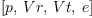(1)
Type: List(OrderedVariableList([p,Vr,Vt,e]))
fricas
eq1a:=((-Vr^3+Vr^2)*Vt+Vr^3-Vr^2)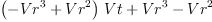(2)
Type: Polynomial(Integer)
fricas
eq1:=((-Vr^3+Vr^2)*Vt+Vr^3-Vr^2)*R*p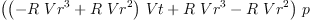(3)
Type: Polynomial(Integer)
fricas
eq2:=-Vr*Vt^3+Vr*Vt^2-Vr*Vt+Vr*p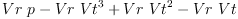(4)
Type: Polynomial(Integer)
fricas
eq3:=(R*Vr*Vt+R*Vr)*e+((R-1)*Vr+1)*Vt-R*Vr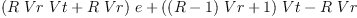(5)
Type: Polynomial(Integer)
fricas
eq4:=-r^2+(Vt^2)+(Vr^2)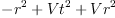(6)
Type: Polynomial(Integer)
fricas
Eqlista:=[eq1a,eq2,eq3,eq4]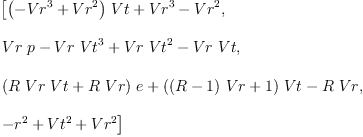(7)
Type: List(Polynomial(Integer))
fricas
Eqlist:=[eq1,eq2,eq3,eq4]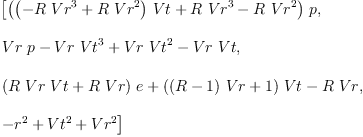(8)
Type: List(Polynomial(Integer))
fricas
solve(Eqlista,list)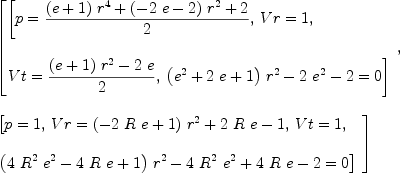(9)
Type: List(List(Equation(Fraction(Polynomial(Integer)))))

  solve(Eqlist,list)


Maple result --billpage, Wed, 01 Feb 2006 22:54:20 -0600 reply
It seems to me that Axiom does not really give a complete solution. Compare this to the result using Maple:
 > solve(Eqlista,list);
2
-%1 - 1 + r
[[p = 1, Vr = %1, Vt = 1, e = 1/2 ------------],
2
R (-1 + r )

2
2           2                         -2 %1 + r
[p = r  %1 + 1 - r , Vr = 1, Vt = %1, e = - ----------]]
2
r  - 2

2         2
%1 := RootOf(-r  + 1 + _Z )


This looks the same as FriCAS answer.

Maple seems to agree that the 2nd problem is considerably more complex than the first, but finds the solution in only a few seconds:

 > solve(Eqlist,list);
[[p = 0, Vr = -r, Vt = 0, e = 1], [p = 0, Vr = r, Vt = 0, e = 1], [

p = 0, Vr = %3, Vt = %2,

2 %3 R %2 - %3 %2 - %3 R - %3 + %2 + 1
e = -1/3 --------------------------------------],
%3 R

2
-%1 - 1 + r
[p = 1, Vr = %1, Vt = 1, e = 1/2 ------------],
2
R (-1 + r )

2
2           2                         -2 %1 + r
[p = r  %1 + 1 - r , Vr = 1, Vt = %1, e = - ----------]]
2
r  - 2

2         2
%1 := RootOf(-r  + 1 + _Z )

2
%2 := RootOf(_Z  - _Z + 1)

2     2
%3 := RootOf(-r  + _Z  + %2 - 1)


Looks like your right, although I will have to back substitute to be sure. So there is a simpler, at least faster, resolution than the one axiom uses. What is _z ? BTW: I like axioms presentation better than Maple's. Those %1 just seem to obscure the answer. Does Maple have a certificate/trace facility? Does axiom's trace, which I can't do on the windows version, provide the same type of certificate? Since I am doing this for a circuit at work, it might be nice to include this in the Doc's; not necessary. My company is on a ISO9000 kick and throwing some chum in the water, extra paper, might satisfy them. They know I have a critical view of some things, like explaining circuits to HR people.

In Maple's solution _Z is a free variable. RootOf is like zerosOf in Axiom. The %1, %2 symbols denote common subexpressions that Maple decided would simplify printing the output.

So for example:

fricas
s1 := zerosOf(-r^2 + 1 + Z^2, Z)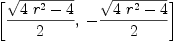(10)
Type: List(Expression(Integer))
fricas
s2 := zerosOf(Z^2 - Z + 1, Z)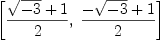(11)
Type: List(Expression(Integer))
fricas
s3a := zerosOf(-r^2 + Z + s2.1 - 1, Z)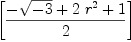(12)
Type: List(Expression(Integer))
fricas
s3b := zerosOf(-r^2 + Z + s2.2 - 1, Z)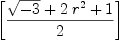(13)
Type: List(Expression(Integer))

As far as I can understand your question ... No neither Maple nor Axiom have any kind of "certificate/trace" facility. But back substituting the answers back into the equations to check the solutions is a good idea in any computer algebra system!

Maybe you could describe in more detail what you mean by a "certificate/trace" facility? What functionality would you expect?

In some Maple programs you can ask for a "proof" (which I think is sometime called a certificate) and it will print out enough intermediate steps to satisfy (some) people. I have been thinking that traceing the subroutine that resolves the S-expressions would give the succesion of simpler, in the grobner well ordering sense, polynomial equations. That should be sufficient. Back substitution vefies the answers, but doesn't prove that you have all of the answers; which in worst-case analysis is needed (you might have overlooked a worst case).

Ray

I want to modify groebsol.spad like so:
   -- general solve, gives an error if the system not 0-dimensional
groebSolve(leq: L DPoly,lvar:L OV) : L L DPoly ==
-- lnp:=[dmpToHdmp(f) for f in leq]
-- replacement
lnp:=[(f :: HDMP(lvar,POLY INT)) for f in leq]

Which I suspect is more correct.

While the statement seems to work from the console on: L DistributedMultivariatePolynomial
The compiler objects?

Some hints/assistance would be appreciated.

Ray


Well I will try again:
  I am trying to modify groabsol.spad like so
groebSolve(leq: L DPoly,lvar:L OV) : L L DPoly ==
-- lnp:=[dmpToHdmp(f) for f in leq]
lnp:=[(f :: HDMP(lvar,POLY INT)) for f in leq]
Which I believe is more correct
HDMP works on L DMP from the console but the compiler objects


Help would be appreciated.

So you are saying that f should be converted to a HDMP using the variables given by lvar rather than lv?

(PolToPol(lv, F) is importet in GROEBSOL)

Note that you cannot simply convert a DMP to a HDMP using the :: notation for coerce, since there is no operation coerce with signature DMP -> HDMP. This is the reason why there is a package polToPol, although I find this a great nuisance. I think that the real reason behind this is that you cannot "extend" domains in Axiom, as you can in Aldor.

In the interpreter you can, since it knows about PolToPol.

Thus, if your analysis is correct, you would have to write:

  lnp:=[dmpToHdmp(f)$PolToPol(lvar,POLY INT) for f in leq]  Martin groebsol.spad compile --rrogers, Mon, 13 Feb 2006 07:06:20 -0600 reply Maritn:  Thanks for the help. I have made some progress; but I haven't made a firm determination yet. I think a practical, and perhaps design problem, is a lack a discrimination between variables and parameters in the simultanious polynomial solver. On a practical side it wastes time in an algorithm that is prone to wandering off for a long time. On the design side not having the equations ordered properly might confuse "groebner" and "groebSolve". I haven't found that part of the coding self evident. I take it that you think that dmpToHdmp orders by lv? That would be good, I haven't looked at it yet. Since I didn't assign lv initially in the code, I didn't find out about it till later. How do you access lv? I looked through the books but didn't find out how; I'm back to looking at cdr/car.  Ray I'm a lamer on groebner bases... However: Line 78 of groebsol.spad says:  import PolToPol(lv,F)  which has the effect that dmpToHdmp(f) is the same as dmpToHdmp(f)$PolToPol(lv,F). Here, lv is a parameter to the package GROEBSOL as you can see in line 37 of groebsol.spad, that reads:

  GroebnerSolve(lv,F,R) : C == T


I don't really understand your question about accessing lv. If you use groebSolve from the interpreter, you would have to supply the value to lv as parameter to GroebnerSolve, since you have to package call groebSolve... To access lv, for example, you could modify groebSolve as follows:

  groebSolve(leq: L DPoly,lvar:L OV) : L L DPoly ==
output(lv::OutputForm)\$OutputPackage
lnp:=[dmpToHdmp(f) for f in leq]


and it will spit out the value of lv every time groebSolve is called...

Questions:

• did you find a bug (i.e., wrong result), or did you experience slow response, or what are you trying to accomplish * could you PLEASE post on axiom-developer. It is difficult for me to follow here. And the discussions here are not archived!

Martin

 Subject: (replying)   Be Bold !! ( 14 subscribers )